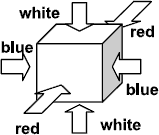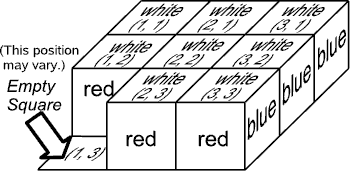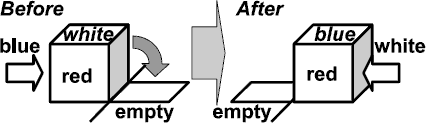Online JudgeProblem SetAuthorsOnline ContestsUser
Web Board
F.A.Qs
Statistical Charts
Problems
Submit Problem
Online Status
Prob.ID:
Register
Authors ranklist
Current Contest
Past Contests
Scheduled Contests
Award Contest
Register
Language:
Cubic Eight-Puzzle
 Time Limit: 5000MS Memory Limit: 65536K Total Submissions: 2016 Accepted: 676

Description

Let’s play a puzzle using eight cubes placed on a 3 × 3 board leaving one empty square.

Faces of cubes are painted with three colors. As a puzzle step, you can roll one of the cubes to a adjacent empty square. Your goal is to make the specified color pattern visible from above by a number of such steps.

The rules of this puzzle are as follows.

1. Coloring of Cubes: All the cubes area colored in the same way as shown in Figure 1. The opposite faces have the same color.Figure 1: Coloring of a cube

2. Initial Board State: Eight cubes are placed on the 3 × 3 board leaving one empty square. All the cubes have the same orientation as shown in Figure 2. As shown in the figure, squares on the board are given x and y coordinates, (1, 1), (1, 2), …, and (3, 3). The position of the initially empty square may vary.Figure 2: Initial board state

3. Rolling Cubes: At each step, we can choose one of the cubes adjacent to the empty square and roll it into the empty square, leaving the original position empty. Figure 3 shows an example.Figure 3: Rolling a cube

4. Goal: The goal of this puzzle is to arrange the cubes so that their top faces form the specified color pattern by a number of cube rolling steps described above.

Your task is to write a program that finds the minimum number of steps required to make the specified color pattern from the given initial state.

Input

The input is a sequence of datasets. The end of the input is indicated by a line containing two zeros separated by a space. The number of datasets is less than 16. Each dataset is formatted as follows.

 x y F11 F21 F31 F12 F22 F32 F13 F23 F33

The first line contains two integers x and y separated by a space, indicating the position (x, y) of the initially empty square. The values of x and y are 1, 2, or 3.

The following three lines specify the color pattern to make. Each line contains three characters F1j, F2j, and F3j, separated by a space. Character Fij indicates the top color of the cube, if any, at the position (i, j) as follows:

`B:` Blue,

`W:` White,

`R:` Red,

`E:` the square is Empty.

There is exactly one ‘`E`’ character in each dataset.

Output

For each dataset, output the minimum number of steps to achieve the goal, when the goal can be reached within 30 steps. Otherwise, output “`-1`” for the dataset.

Sample Input

```1 2
W W W
E W W
W W W
2 1
R B W
R W W
E W W
3 3
W B W
B R E
R B R
3 3
B W R
B W R
B E R
2 1
B B B
B R B
B R E
1 1
R R R
W W W
R R E
2 1
R R R
B W B
R R E
3 2
R R R
W E W
R R R
0 0```

Sample Output

```0
3
13
23
29
30
-1
-1```

Source

[Submit]   [Go Back]   [Status]   [Discuss]Home PageGo BackTo top